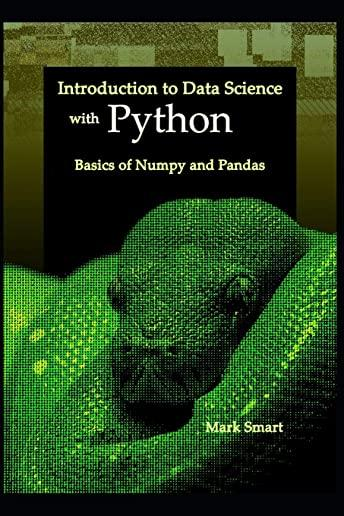### Introduction to Data Science with Python: Basics of Numpy and Pandas### Huge savings for students

Each student receives a 50% discount off of most books in the HSG Book Store. During class, please ask the instructor about purchase details.
 List Price: \$9.38 Price: \$4.69 You Save: \$4.69
2This book is a guide for you on how to use Pandas and Numpy in Python programming language for data analysis. The author begins by helping you familiarize yourself with the basics of data science, Numpy and Pandas. You are guided on how to work with Numpy arrays and how to manipulate them. The various operations that you can perform on your data via the Pandas library have been discussed. You will also know how to create various data structures in Pandas for data storage. Data from the environment is dirty. The process of cleaning such data has been discussed. This involves handling outliers, missing values etc. The author guides you on how to work with data in various types of storage formats. Examples include MS Excel, CSV files, JSON, etc. You are also guided on how to calculate various measures for your data. The process of visualizing data has been explored in detail. About this book:
• Getting Started with Python for Data Science
• Working with Numpy
• Working with Pandas
• Cleansing Data
• Working with CSV Data
• Working with XLS Data
• Data Wrangling
• Measures of Central Tendency
• Calculating Variance
• Normal Distribution
• Working with JSON Data
• Data Visualization
Tags: data science with python, python, pandas programming, numpy, pandas, pandas python, pandas in python, numpy in python, numpy python, numpy pandas, data science, ms excel books, json, python for data science, pivot tables, excel pivot tables, data visualisation, data visualisation python, data visualisation for dummies, data visualisation excel, algorithms for data science.
Independently Published